# Mathematics Materials

All math units are available for purchase from Prufrock Press. We encourage you to purchase the materials directly from our publisher, and you can do so by clicking on each book’s title to visit Prufrock’s site.

Thinking Like a Mathematician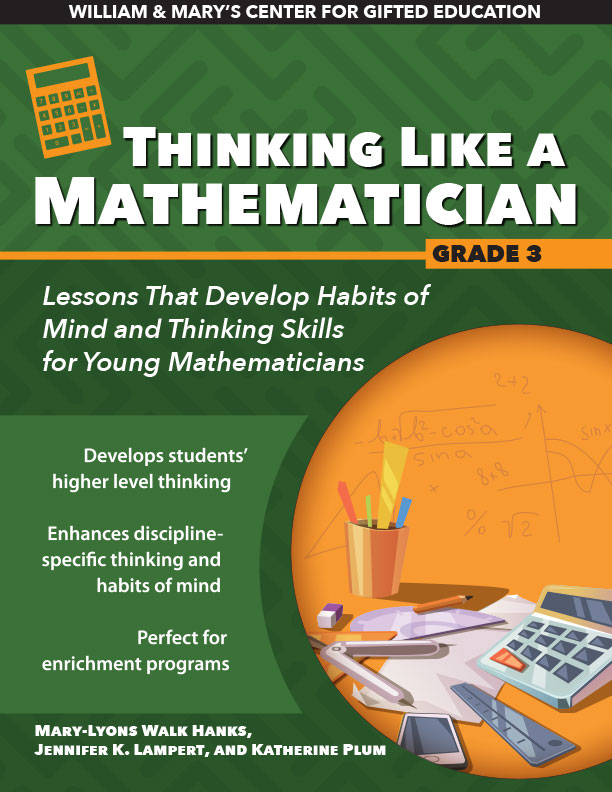Thinking Like a Mathematician focuses on high-interest, career-related topics in the elementary curriculum related to mathematics. Students will explore interdisciplinary content, foster creativity, and develop higher order thinking skills with activities aligned to relevant content area standards. Students will engage in exploration activities, complete mathematical challenges, and then apply what they have learned by making real-world connections.

######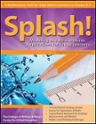Splash! is a mathematics unit for high-ability learners in grades K–1 focusing on concepts related to linear measurement, the creativity elements of fluency and flexibility, and the overarching, interdisciplinary concept of models. The unit consists of 13 lessons centered on the idea of designing a community pool. Students examine the question of why we measure, the importance of accuracy in measurement, and the various units and tools of measurement.

###### Spatial Reasoning

Spark the visual learning of students in grades 2–4 with Spatial Reasoning, a mathematics unit for high-ability learners. Gifted students demonstrate an advanced aptitude for spatial reasoning at early ages, and they require more complex lessons than what the standard curriculum provides. This field-tested unit approaches spatial reasoning through one-dimensional, two-dimensional, and three-dimensional tasks that will engage students.

###### Polygons Galore!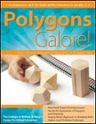Polygons Galore!
is a mathematics unit for high-ability learners in grades 3–5 focusing on 2-D and 3-D components of geometry by exploring polygons and polyhedra and their properties. The van Hiele levels of geometric understanding provide conceptual underpinnings for unit activities. The unit consists of nine lessons that include student discovery of properties of polygons and polyhedra, investigations for finding areas of triangles and quadrilaterals, study of the Platonic solids, and real-world applications of polygons and polyhedra.

###### Beyond Base Ten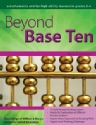Looking for a way to challenge your gifted students grades 3–6? Look no further! Beyond Base Ten investigates the concept of place value and the representation of numbers by using place value and non-place-value systems. Number bases other than Base Ten are featured, especially through historical contexts of early civilizations that developed number systems different from the one we use today.

###### Moving Through Dimensions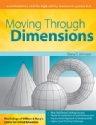Moving Through Dimensions approaches spatial reasoning through one-dimensional, two-dimensional, and  three-dimensional tasks designed for students in grades 6–8. The unit also asks students to explore the transition between dimensions and representations of three-dimensional objects in two dimensions. This book includes pre- and post-assessments, multiple reproducible materials, and lessons based on NCTM standards.
###### The Math Curriculum for Gifted Students Series

provides resources for gifted and advanced learners in grades 3 - 6 to extend their learning whether in a formal gifted and talented program, a special advanced mathematics class, a pull-out math enrichment program, or within a general education classroom to differentiate instructions for individuals, groups, or clusters of students. Complete sets are available for each grade level. A complete set includes the teacher's guide and 5 copies of each companion student workbook.

###### Grade 3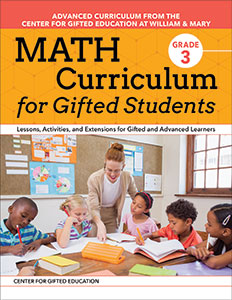The 21 lessons cover mathematics content for grade 3 and are divided into five sections: number and operations in Base Ten, operations and algebraic thinking, number and operations–fractions, measurement and data, and geometry.

###### Grade 4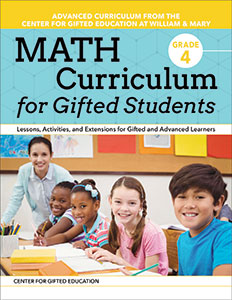The 24 lessons cover mathematics content for grade 4 and are divided into five sections: number and operations in Base Ten, operations and algebraic thinking, number and operations–fractions, measurement and data, and geometry.

###### Grade 5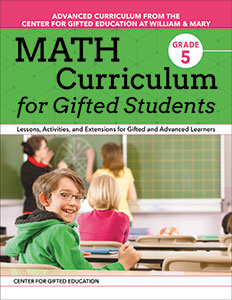The 20 lessons cover mathematics content for grade 5 and are divided into five sections: number and operations in Base Ten, operations and algebraic thinking, number and operations–fractions, measurement and data, and geometry.

###### Grade 6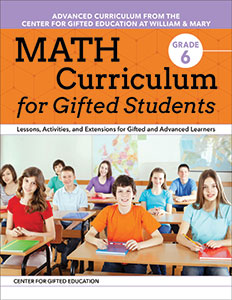The 21 lessons cover mathematics content for grade 6 and are divided into five sections: ratios and proportional relationships, the number system, expressions and equations, geometry, and statistics and probability.